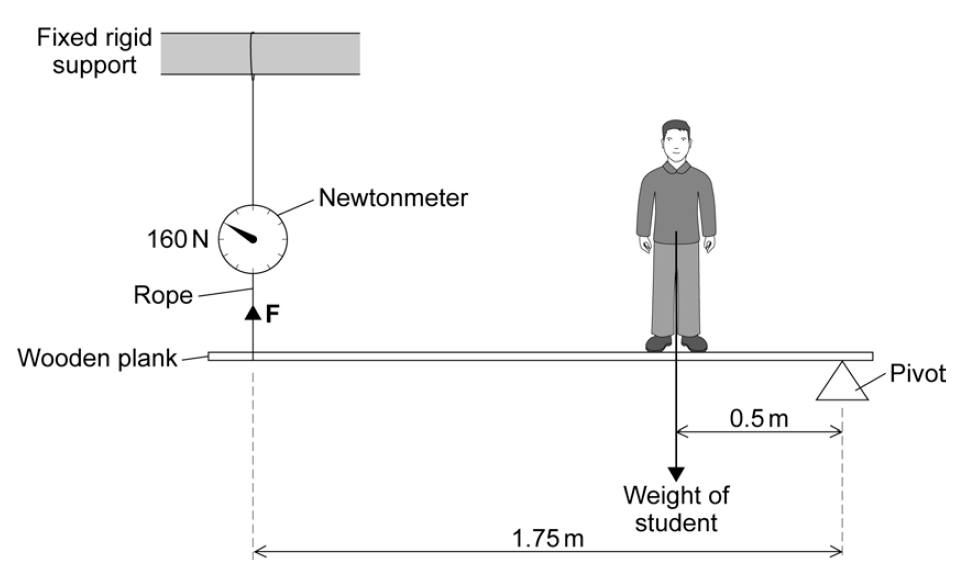# Moments – Question 1

A student wants to weigh himself but the only balance available is a newtonmeter that measures up to 200 newtons.

The diagram shows how the student solved the problem using moments.(a) Use the information in the diagram to calculate the weight of the student given by this method.

Write down the equations you use, and then show clearly how you work out your answer and give the unit.Weight = .............................
(5 marks)

(b) Even though all the measurements are accurate the student’s weight obtained by this method is inaccurate.

Explain why.(2 marks)

[expand title="How did you do? Click to show the mark scheme" startwrap="" endwrap=""][/expand]

From Q8 P3 AQA Specimen Paper (Higher)